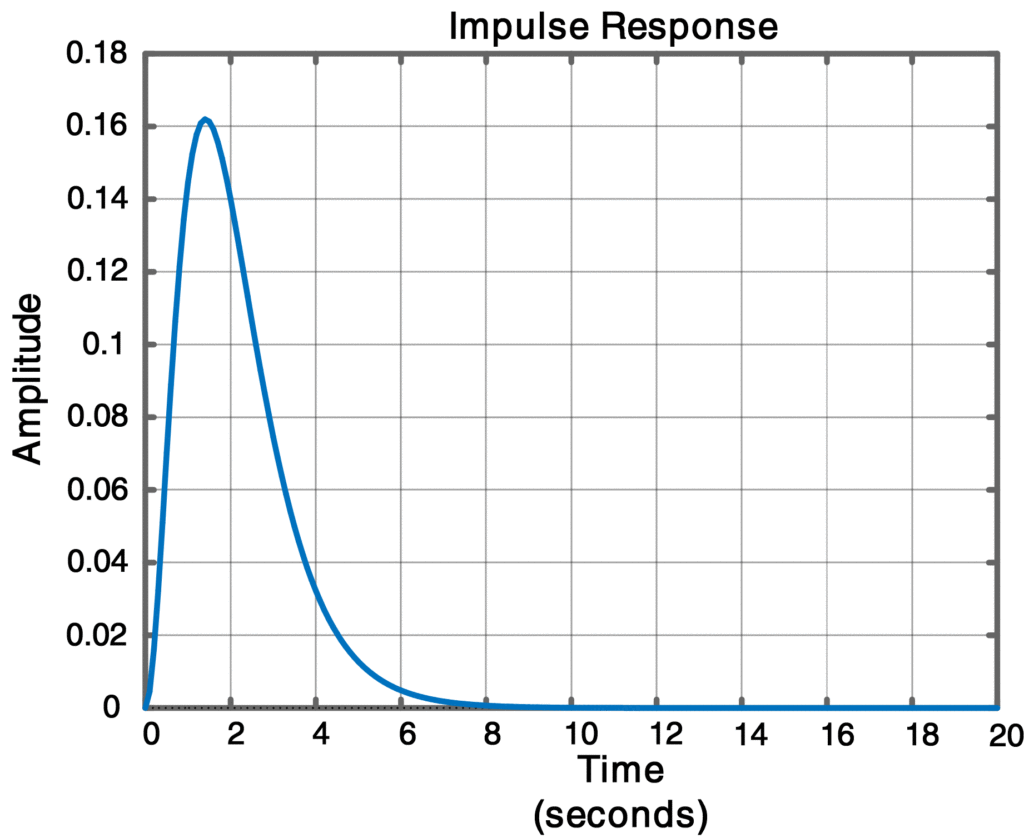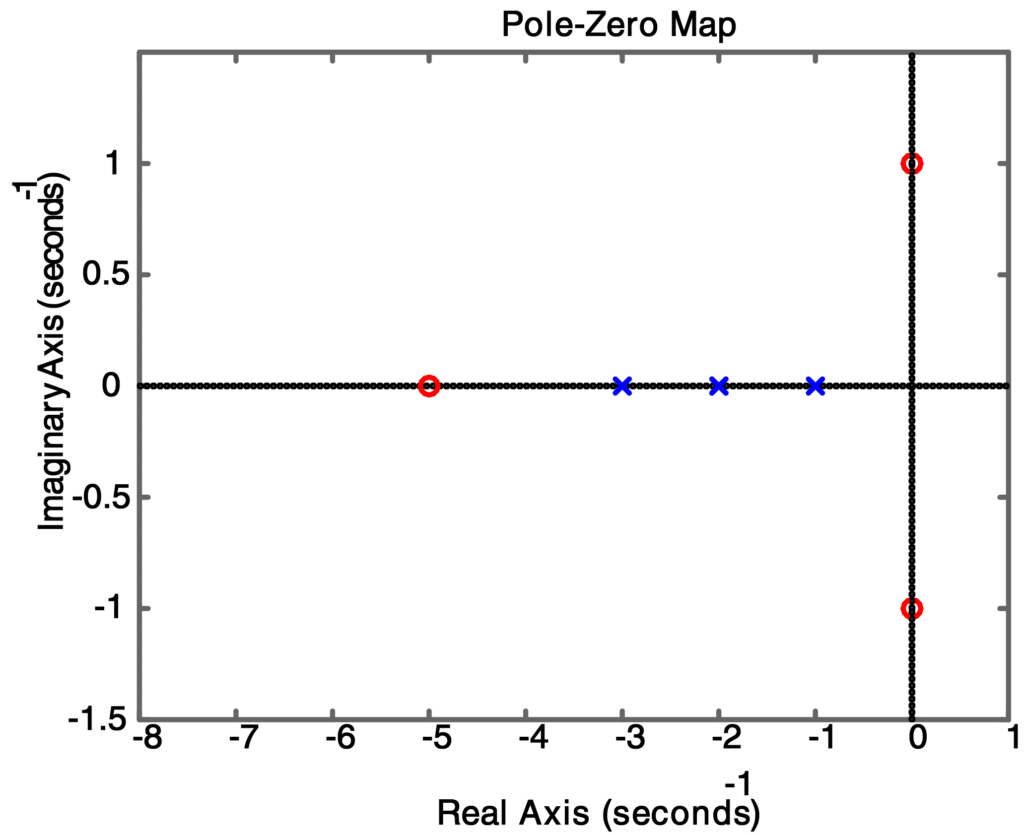Home / Control with Matlab / Effect of Zeros on System Response

# Effect of Zeros on System Response

Want create site? Find Free WordPress Themes and plugins.

The zeros zj of G(s) do not affect the system stability. However, they do affect the amplitudes of the mode functions in the system response and can block the transmission of certain input signals. In general, to assess the Impact of the zeros on the amplitude of the mode functions, a partial-fraction expansion is performed on the Laplace transform of the output signal, and the residue for each mode function is computed. However, certain properties of zeros can be readily illustrated in the time domain as shown in the following Example.

Effect of Zeros on System Response with Example

Find the time response of the output y (t) of the system in the factored form

$G(s)=\frac{(s+5)({{s}^{2}}+1)}{(s+1){{(s+2)}^{2}}(s+3)}$

Subject to the input u (t) = sin t for t≥0. Does y (t) contain any sinusoidal component? Also, draw a plot of the poles and zeros in the s-plane.

Solution

In MATLAB Script 8, we deﬁne zz = [-5; i; -i], pp = [-1; -2; -2; -3] and kk = 1. The TF form of G (s) is obtained as [numG, denG] = zp2tf (zz, pp, kk), yielding numG = [1 5 1 5] and denG = [1 8 23 28 12].We generate the time vector t = [0:0.1:20]’ and the input vector u = sin (t). Then the command lsim (numG, denG, u, t) is used to simulate the time response y (t), which is shown in Figure 1. Note that y (t) consists solely of the modes of G(s) and does not exhibit any sinusoidal component due to the input u (t). To understand this phenomenon, we obtain the Laplace transform of u (t) as

$U(s)=L\left\{ u(t) \right\}=L\left\{ sin(t) \right\}=\frac{1}{{{s}^{2}}+1}$

Then the Laplace transform of the output y (t) is the product

$Y(s)=G(s)U(s)=\frac{(s+5)}{(s+1){{(s+2)}^{2}}(s+3)}$

Note that the zeros of G(s) at s = ±j1 cancel the poles of U (s). The response y (t) can be found from the command impulse ([1 5], denG, t) and will be identical to the response obtained from the lsim command. The MATLAB commands in Script 8 will compute the time response and make the plots as shown in figure 1 and 2.

## Matlab Code to Compute Output Impulse Response and Pole-Zero Plot


%Script 8: Matlab Code to compute output Impulse Response y(t)

clear all;close all;clc

zz = [-5; 1i; -1i];   % Zeros of G(s)

pp = [-1; -2; -2; -3]; % Poles of G(s)

kk = 1;  % Gain of G(s)

% Generate G(s) in Transfer Function(TF) form

[numG,denG] = zp2tf(zz,pp,kk);% convert G(s) to TF form

t = 0:0.1:20;% generate time array

u = sin(t); % generate input signal

lsim(numG,denG,u,t) % simulate system response y(t)

impulse([1 5],denG,t) % impulse response (of y(t)) without zeros at ±j

pzmap(numG,denG)% plot poles and zeros of G(s) in s—plan



ResultsFig.1: Impulse Response of Transfer FunctionFig.2: Pole Zero Plot of Transfer Function

Did you find apk for android? You can find new Free Android Games and apps.